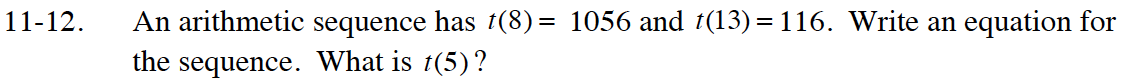### Home > CC4 > Chapter 11 > Lesson 11.1.1 > Problem11-12

11-12.

An arithmetic sequence has t(8) = 1056 and t(13) = 116. Write an equation for the sequence. What is t(5)? Homework Help ✎The general equation for an arithmetic sequence is t(n) = mn + b.

Use t(8) = 1056 to write an equation.
t(8) = m(8) + b or 1056 = 8m + b

Use t(13) = 116 to write another equation.

Solve the system of equations you wrote for m and b.

t(n) = −188n + 2560

To find t(5), substitute 5 for n in the equation that you wrote.